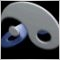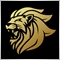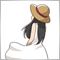# RSI EA code help - page 2341

Marco vd Heijden:

YES

//+------------------------------------------------------------------+
//|                                                          RSI.mq4 |
//|                               Copyright © 2016, Хлыстов Владимир |
//|                                                cmillion@narod.ru |
//+------------------------------------------------------------------+
#property copyright "Copyright © 2016, Хлыстов Владимир"
#property link      "cmillion@narod.ru"
#property strict
#property description "советник по RSI"
#property description "sell при пересечение сверху вниз 70 и на buy снизу вверх 30"
#property description "стопы и тейки можно выстовить в настройках советника"
//--------------------------------------------------------------------
extern int     period_RSI           = 14,
stoploss             = 100,
takeprofit           = 200,
slippage             = 10,
buy_level            = 30,
sell_level           = 70,
Magic                = 777;
extern double  Lot                  = 0.1;
//--------------------------------------------------------------------
void OnTick()
{
for (int i=0; i<OrdersTotal(); i++)
if (OrderSelect(i,SELECT_BY_POS,MODE_TRADES))
if (OrderSymbol()==Symbol() && Magic==OrderMagicNumber()) return;
double RSI0  = iRSI(NULL,0,period_RSI,PRICE_OPEN,N);
double RSI1  = iRSI(NULL,0,period_RSI,PRICE_OPEN,N);
double SL=0,TP=0;
if (RSI0 > buy_level && RSI1 < buy_level)
{
if (takeprofit!=0) TP  = NormalizeDouble(Ask + takeprofit*Point,Digits);
if (stoploss!=0)   SL  = NormalizeDouble(Ask - stoploss*  Point,Digits);
if (OrderSend(Symbol(),OP_BUY, Lot,NormalizeDouble(Ask,Digits),slippage,SL,TP,NULL,Magic)==-1) Print(GetLastError());
}
if (RSI0 < sell_level && RSI1 > sell_level)
{
if (takeprofit!=0) TP = NormalizeDouble(Bid - takeprofit*Point,Digits);
if (stoploss!=0)   SL = NormalizeDouble(Bid + stoploss*  Point,Digits);
if (OrderSend(Symbol(),OP_SELL,Lot,NormalizeDouble(Bid,Digits),slippage,SL,TP,NULL,Magic)==-1) Print(GetLastError());
}
}
//--------------------------------------------------------------------

Great to replace 0 and 1 with N :)

But N is changing everytime on different charts and different currency pairs.

how to do we determine N between two RSI events in the code ?Moderator
10735

You would have to define N first.106

Hello guys ,i need some help for coding:

i used RSI_Extremums_Sample indicator and double_smoothed_stochastic indicator in my expert .

but when i put in strategy tester don't work and my mt4 platform hanged . can anyone help me why is that ?

this is my code to get position :

```string position()
{
if(((iCustom(Symbol(),PERIOD_CURRENT,"double_smoothed_stochastic",0,1)>iCustom(Symbol(),PERIOD_CURRENT,"double_smoothed_stochastic",0,2))&&
(iCustom(Symbol(),PERIOD_CURRENT,"double_smoothed_stochastic",0,1)>20)&&
(iCustom(Symbol(),PERIOD_CURRENT,"RSI_Extremums_Sample",0,1)>iCustom(Symbol(),PERIOD_CURRENT,"RSI_Extremums_Sample",0,2))&&
(iCustom(Symbol(),PERIOD_CURRENT,"RSI_Extremums_Sample",0,1)<80)))
return("buy");
if((iCustom(Symbol(),PERIOD_CURRENT,"double_smoothed_stochastic",0,1)<iCustom(Symbol(),PERIOD_CURRENT,"double_smoothed_stochastic",0,2))&&
(iCustom(Symbol(),PERIOD_CURRENT,"double_smoothed_stochastic",0,1)<80)&&
(iCustom(Symbol(),PERIOD_CURRENT,"RSI_Extremums_Sample",0,1)<iCustom(Symbol(),PERIOD_CURRENT,"RSI_Extremums_Sample",0,2))&&
(iCustom(Symbol(),PERIOD_CURRENT,"RSI_Extremums_Sample",0,1)>20))
return("sell");
else
return("no signal");
}```

i don't have any error in journal but test don't go forward and stop for hours ....

sorry about my poor english;

Files:
Untitled.png 251 kb
Untitled2.png 280 kb1324

milad najjari:

Hello guys ,i need some help for coding:

i used RSI_Extremums_Sample indicator and double_smoothed_stochastic indicator in my expert .

but when i put in strategy tester don't work and my mt4 platform hanged . can anyone help me why is that ?

this is my code to get position :

i don't have any error in journal but test don't go forward and stop for hours ....

sorry about my poor english;

You need this indicator ;

```      Values[i] = NormalizeDouble(iCustom(Symbol(),0,"Cycle_KROUFR_version",FastMA,SlowMA,Crosses ,0, i), DigitsUsed);
```106

ffoorr:

You need this indicator ;

i use this indicator and change code to this , but problem not solved , strategy tester don't movie

```string position()
{
if((iCustom(Symbol(),PERIOD_CURRENT,"double_smoothed_stochastic",0,1)>iCustom(Symbol(),PERIOD_CURRENT,"double_smoothed_stochastic",0,2))&&
(iCustom(Symbol(),PERIOD_CURRENT,"double_smoothed_stochastic",0,1)>20)&&
(NormalizeDouble(iCustom(Symbol(),PERIOD_CURRENT,"Cycle_KROUFR_version",12,24,50,false,0,1),5)>
NormalizeDouble(iCustom(Symbol(),PERIOD_CURRENT,"Cycle_KROUFR_version",12,24,50,false,0,2),5))&&
(NormalizeDouble(iCustom(Symbol(),PERIOD_CURRENT,"Cycle_KROUFR_version",12,24,50,false,0,1),5)<80))
return("buy");
if((iCustom(Symbol(),PERIOD_CURRENT,"double_smoothed_stochastic",0,1)<iCustom(Symbol(),PERIOD_CURRENT,"double_smoothed_stochastic",0,2))&&
(iCustom(Symbol(),PERIOD_CURRENT,"double_smoothed_stochastic",0,1)<80)&&
(NormalizeDouble(iCustom(Symbol(),PERIOD_CURRENT,"Cycle_KROUFR_version",12,24,50,false,0,1),5)<
NormalizeDouble(iCustom(Symbol(),PERIOD_CURRENT,"Cycle_KROUFR_version",12,24,50,false,0,2),5))&&
(NormalizeDouble(iCustom(Symbol(),PERIOD_CURRENT,"Cycle_KROUFR_version",12,24,50,false,0,1),5)>20))
return("sell");
else
return("no signal");
}```

or

```string position()
{
if((iCustom(Symbol(),PERIOD_CURRENT,"double_smoothed_stochastic",0,1)>iCustom(Symbol(),PERIOD_CURRENT,"double_smoothed_stochastic",0,2))&&
(iCustom(Symbol(),PERIOD_CURRENT,"double_smoothed_stochastic",0,1)>20)&&
(NormalizeDouble(iCustom(Symbol(),PERIOD_CURRENT,"Cycle_KROUFR_version",12,24,50,0,1),5)>
NormalizeDouble(iCustom(Symbol(),PERIOD_CURRENT,"Cycle_KROUFR_version",12,24,50,0,2),5))&&
(NormalizeDouble(iCustom(Symbol(),PERIOD_CURRENT,"Cycle_KROUFR_version",12,24,50,0,1),5)<80))
return("buy");
if((iCustom(Symbol(),PERIOD_CURRENT,"double_smoothed_stochastic",0,1)<iCustom(Symbol(),PERIOD_CURRENT,"double_smoothed_stochastic",0,2))&&
(iCustom(Symbol(),PERIOD_CURRENT,"double_smoothed_stochastic",0,1)<80)&&
(NormalizeDouble(iCustom(Symbol(),PERIOD_CURRENT,"Cycle_KROUFR_version",12,24,50,0,1),5)<
NormalizeDouble(iCustom(Symbol(),PERIOD_CURRENT,"Cycle_KROUFR_version",12,24,50,0,2),5))&&
(NormalizeDouble(iCustom(Symbol(),PERIOD_CURRENT,"Cycle_KROUFR_version",12,24,50,0,1),5)>20))
return("sell");
else
return("no signal");
}```

i tested two code but both of them have same problem.

Files:441

milad najjari:

i used RSI_Extremums_Sample indicator and double_smoothed_stochastic indicator in my expert .

but when i put in strategy tester don't work and my mt4 platform hanged . can anyone help me why is that ?

An amateur writes such a complicated program. Write a more comprehensible one.

```string position()
{
double a = iCustom(Symbol(),PERIOD_CURRENT,"double_smoothed_stochastic",0,1);
double b = iCustom(Symbol(),PERIOD_CURRENT,"double_smoothed_stochastic",0,2);
double c = iCustom(Symbol(),PERIOD_CURRENT,"double_smoothed_stochastic",0,1);
double d = iCustom(Symbol(),PERIOD_CURRENT,"RSI_Extremums_Sample",0,1);
double e = iCustom(Symbol(),PERIOD_CURRENT,"RSI_Extremums_Sample",0,2);
double f = iCustom(Symbol(),PERIOD_CURRENT,"RSI_Extremums_Sample",0,1);

double g = iCustom(Symbol(),PERIOD_CURRENT,"double_smoothed_stochastic",0,1);
double h = iCustom(Symbol(),PERIOD_CURRENT,"double_smoothed_stochastic",0,2);
double i = iCustom(Symbol(),PERIOD_CURRENT,"double_smoothed_stochastic",0,1);
double j = iCustom(Symbol(),PERIOD_CURRENT,"RSI_Extremums_Sample",0,1);
double k = iCustom(Symbol(),PERIOD_CURRENT,"RSI_Extremums_Sample",0,2);
double l = iCustom(Symbol(),PERIOD_CURRENT,"RSI_Extremums_Sample",0,1);

if ((a > b) && (c > 20) && (d > e) && (f < 80))
return("buy");
else if((g < h) && (i < 80) && (j < k) && (l > 20))
return("sell");
else
return("no signal");
}```

Since "RSI_Extremums_Sample" calls "Cycle_KROUFR_version" with iCustom, these two and "double_smoothed_stochastic" are required.

It worked fine on my PC.106

Naguisa Unada:

An amateur writes such a complicated program. Write a more comprehensible one.

Since "RSI_Extremums_Sample" calls "Cycle_KROUFR_version" with iCustom, these two and "double_smoothed_stochastic" are required.

It worked fine on my PC.

your code has that problem too in my computer .....
To add comments, please log in or register